Custom SearchINDETERMINATE FORMS When the value of a limit is obtained by substitution and it assumes any of the following forms, another method for finding the limit must be used:These are called indeterminate forms. There are many methods of evaluating indeterminate forms. Two methods of evaluating indeterminate forms are (1) factoring and (2) division of the numerator and denominator by powers of the variable. Sometimes factoring will resolve an indeterminate form. EXAMPLE: Find the limit of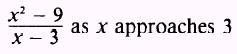SOLUTION: By substitution we find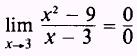which is an indeterminate form and is therefore excluded as a possible limit. We must now search for a method to find the limit. Factoring is attempted, which results in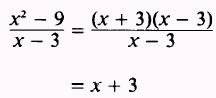so thatand we have a determinate limit of 6. Another indeterminate form is often met when we try to find the limit of a function as the independent variable approaches infinity. EXAMPLE: Find the limit of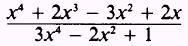SOLUTION: If we let x approach infinity -in the original expression, the result will bewhich must be excluded as an indeterminate form. However, if we divide both numerator and denominator by x4, we obtain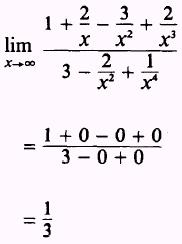and we have a determinate limit of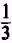. PRACTICE PROBLEMS: Find the limit of the following:ANSWERS: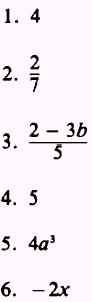Integrated Publishing, Inc. - A (SDVOSB) Service Disabled Veteran Owned Small Business